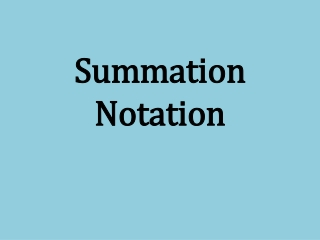DownloadDownload PresentationSummation Notation

# Summation Notation

Télécharger la présentation## Summation Notation

- - - - - - - - - - - - - - - - - - - - - - - - - - - E N D - - - - - - - - - - - - - - - - - - - - - - - - - - -
##### Presentation Transcript

1. Summation Notation

2. Summation notation:a way to show the operation of adding a series of values related by an algebraic expression or formula. The symbol for summation is the Greek letter Sigma, S. Upper limit Algebraic expression The 20th partial sum of the arithmetic sequence: 2, 7, 12, 17,… would be represented as Variable Lower limit Explicit formula of the sequence

3. You can still use the partial sum formulas to find the answer to these, but you have to make sure you recognize if the expression represents an arithmetic or geometric sequence. 1) 2)

4. For each sequence below: • Determine if it is arithmetic or geometric. • Identify the common difference or ratio • Write each in summation notation for the 16th partial sum of each sequence. • 1) 2) • Arithmetic • d = 8 • Geometric • r = 5

5. Infinite Sequences & Series Convergent and Divergent Sequences

6. Some infinite sequences never approach a unique number and continue forever. These sequences are called a divergent sequences Arithmetic sequence 5, 8, 11, 14, … Geometric sequence 2, 4, 8, 16, … What conclusion can you make about the type of sequences that are convergent?

7. Convergent and Divergent Sequences Some infinite sequences approach a unique number. (Consider this an asymptote). When the infinite sequence approaches a unique number, the sequence is a convergent sequence Example: The geometric sequence 8, 2, ½, , … What value do the terms appear to be getting closer to as the sequence continues?

8. If given a sequence, how can you tell if it is a convergent or divergent sequence? • Make a list of the first 4 – 5 terms of the sequence and observe the pattern. • Create a graph of the sequence and observe the trend of the points. Determine if each sequence is convergent or divergent. 1) an = -3n+ 12 2) an = 4 · (½)n-1 3) an = 2 · (-3)n-1 9, 6, 3, 0, -3, … Divergent 4, 2, 1, ½, ¼, … Convergent 2, -6, 18, -54, 162, … Divergent

9. An infinite sum means that you add ALL the terms of infinite sequence. The only type of infinite sequences that can be added are convergent sequences. Formula for the infinite sum of a sequence Remember this is only used on geometric sequences with fractional value for r. -1 < r < 1